• 文章目录多层感知机（multi layer perception, MLP ）反响传播算法（Back propagation） 前一篇文章中我们提到...这就是我们今天要讲到的多层感知机以及用来在多层感知机中用来实现权重迭代的方法—BP（Back propag...


文章目录
多层感知机（multi layer perception, MLP ）反响传播算法（Back propagation）

前一篇文章中我们提到单层感知机网络被局限在解决传统的是非问题，当面对异或这类问题时则显得无能为力，为了解决这一问题，人们通过增加网络层数来提升神经网络解决问题的能力。这就是我们今天要讲到的多层感知机以及用来在多层感知机中用来实现权重迭代的方法—BP（Back propagation）迭代方法。实际上两者混在一起也就是我们常说的BP神经网络。

多层感知机（multi layer perception, MLP ）
多层感知机就是含有至少一个隐藏层的由全连接层组成的神经网络，且在整个神经网络中除了输入层之外，每一层都经过激活函数进行非线性变换。多层感知机的层数和各隐藏层中隐藏单元个数都是超参数。参考下图的单隐藏层的MLP结构：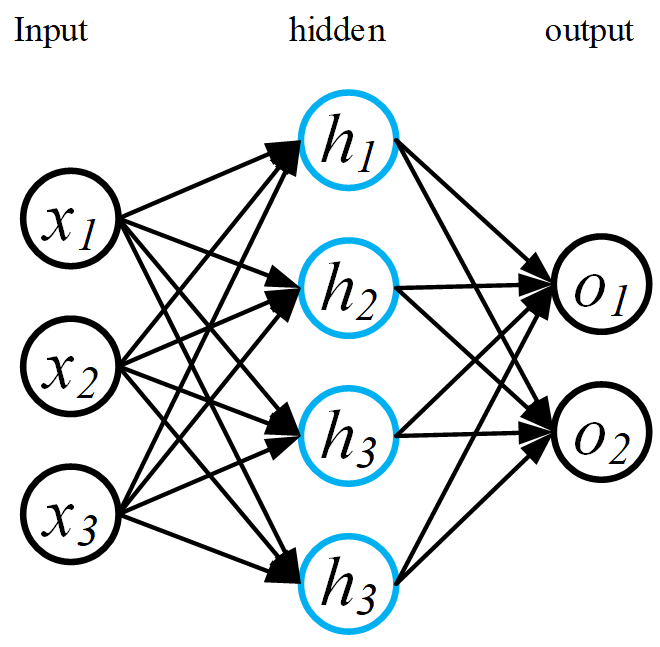反响传播算法（Back propagation）
反响传播算法的基本思想是，利用一次前向过程计算得到的误差，反向传播到每一层的权重上，并实现权重的逐步更新，通过足够多的更新步骤后得到一个较优权重使最终得到的误差能够满足运算标准。 一种最常用的误差反向传递方法是梯度下降法：即通过计算后一层到前一层的运算梯度，使得权重的变化量正比于该梯度的值，以此得到每一层权重的误差。其比例系数就是常说的学习率。
BP 神经网络的相关推导网络上已经有很多，例如戳这，可以详细的了解其推导过程。
下面附上用BP网络实现手写数字识别的代码
#三层神经网络，含有一个隐藏层
#结果大约在84%左右
import math
import pandas as pd
import numpy as np
import matplotlib.pyplot as plt

#读入数据

#数据归一化
dataset /=256.0
dataset = dataset.values
#print (type(dataset))
dataset_label = dataset_label.values

#神经网络配置
dataset_num = len(dataset)
input_num =dataset.shape       #确定输入节点数
out_num = 10
hid_num = 12     #隐藏层节点数
#初始化输入权重矩阵
w12 = 0.2*np.random.random((input_num,hid_num)) - 0.1
# 初始化隐层权矩阵
w23 = 0.2*np.random.random((hid_num, out_num))- 0.1
print(w12.shape)
hid_offset = np.zeros(hid_num)      # 隐层偏置向量
out_offset = np.zeros(out_num)      # 输出层偏置向量
input_learnrate = 0.15              # 输入层权值学习率
hid_learnrate = 0.15                # 隐层学权值习率
err_th = 0.01                       # 学习误差门限

#print(dataset.loc[1:2].shape)

#定义激活函数
def get_act(x):
act_vec = []
for i in x:
act_vec.append(1/(1+math.exp(-i)))
act_vec = np.array(act_vec)
return act_vec

def get_err(e):
return 0.5*np.dot(e,e)

# 训练——可使用err_th与get_err() 配合，提前结束训练过程

for count in range (0,dataset_num):
t_label =  np.zeros(out_num)
t_label[dataset_label[count]] = 1
#前向过程
hid_value = np.dot(dataset[count],w12)
hid_act = get_act(hid_value)
out_value = np.dot(hid_act,w23)
out_act = get_act(out_value)

#反向过程

e = t_label - out_act
out_delta = e*out_act*(1-out_act)
hid_delta = hid_act * (1-hid_act)*np.dot(w23,out_delta)
for i in range(0,out_num):
#更新隐藏层到输出层权向量
w23[:,i] += hid_learnrate * out_delta[i] * hid_act
for i in range (0,hid_num):
#更新输入层到隐藏层权重
w12[:,i] += input_learnrate * hid_delta[i] * dataset[count]

out_offset += hid_learnrate * out_delta
hid_offset += input_learnrate * hid_delta

#测试
test_data /=256.0
test_data = test_data.values

test_label =test_label.values

right = np.zeros(10)
numbers = np.zeros(10)

# 统计测试数据中各个数字的数目
print(test_label.shape)

for i in test_label:
numbers[i] += 1

for count in range(len(test_data)):
hid_value = np.dot(test_data[count],w12) + hid_offset
hid_act = get_act(hid_value)
out_value = np.dot(hid_act,w23) + out_offset
out_act = get_act(out_value)
if np.argmax(out_act) == test_label[count]:
right[test_label[count]] += 1

print (right)
print (numbers)
result = right/numbers
sum = right.sum()
print (result)
print (sum/len(test_data))


本文代码和数据可以在我的github主页上找到。
展开全文python 深度学习 机器学习
• 本文主要介绍了神经网络入门必须要了解的两个算法：感知机和BP神经网络。从人工神经网络的由来到感知机算法的原理、BP算法的原理及推导。


神经网络知识总结
人工神经网络的由来为什么要使用神经网络？从人体神经网络系统到人工神经网络
最简单的神经网络——感知机感知机的构造感知机权值更新的概念和计算感知机的权值更新感知机详细计算过程

增加hidden layer的神经网络——BP神经网络为什么要增加隐层？delta学习规则BP神经网络权值更新的梯度下降法推导过程BP神经网络计算实例
notes：感知机和线性回归、逻辑回归的关联总结python实现——sklearn葡萄酒分类

人工神经网络的由来
为什么要使用神经网络？
我们知道使用线性回归和逻辑回归可以解决线性可分问题，对于有些线性不可分的问题，也可以通过增加多项式来解决，但是对于决策边界较为复杂的情况：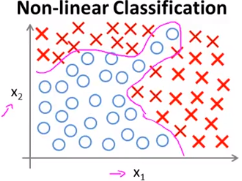此时，往往需要引入较多的多项式，对于变量较多的情况极为复杂，甚至容易出现过拟合。 由于大量数据的涌入和收集(大数据的驱动)我们有了新的算法：人工神经网络（ANN），它的研究是由试图模拟生物神经系统而激发的。
从人体神经网络系统到人工神经网络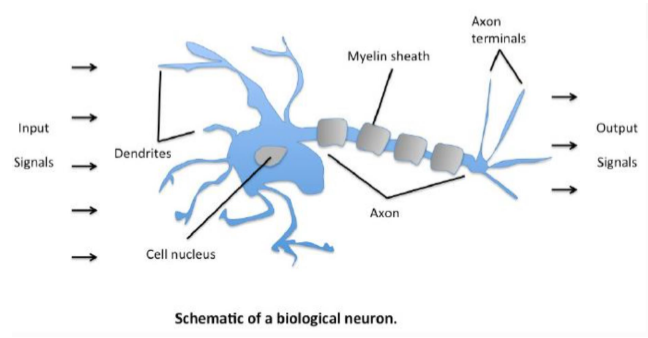人的大脑主要由称为神经元（节点）的神经细胞组成，神经元通过突触的纤维丝连在一起。当神经元受到刺激时，神经脉冲(输入)通过轴突从一个神经元传到另一个神经元。一个神经元的树突连接到其他神经元的轴突，其连接点称为神经建(权重)。 神经学家发现，人的大脑通过在同一个脉冲（输入）反复刺激下改变神经元之间的神经建连接强度（权值）来进行学习。 PS.以一个例子来看人工神经网络的运作方式： 这里借用朱江老师上课所讲的例子——《甄嬛传》高潮部分：滴血认亲 看过甄嬛传的同学应该不会忘了这一集，剧情是相当刺激~内容是这样的，祺嫔张罗一群人来指认甄嬛腹中双生子并非皇上的，而是太医温实初的。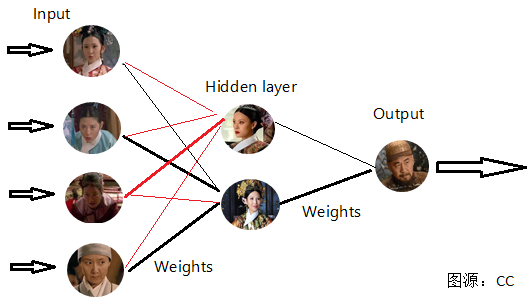(PS.图片为博主原创，转载请注明出处，谢谢！) 如上图，开始有祺嫔、丫鬟佩儿、侍女玢儿、尼姑净白被幕后主使召集起来，分别指认甄嬛腹中并非龙种，此时，这四个人作为信息的输入(input)作为幕后主使的皇后，和当事人甄嬛分别对输入信息加权汇总找出对自己有利的信息，并适当为收集信息“添油加醋”。上图连接线的粗细表示信息的权重(Weights)，作为皇上，自然担心自己孩子血统是否纯正，因此对于皇后观点权重较大,此时皇上作为对全部信息的汇总输出，可能得出结论：孩子不是自己的。但是根据不断的信息涌入和转换，对权重进行调整，最终的结果是皇上认为：孩子是自己的。 以上就是一个人工神经网络传递信息的例子。由上可知，人工神经网络的一些组成是：

{

输

入

I

n

p

u

t

:

x

1

,

x

2

,

.

.

.

,

x

n

(

n

个

特

征

)

权

值

W

e

i

g

h

t

s

:

w

1

,

w

2

,

.

.

.

,

w

3

激

活

函

数

f

(

x

)

[

可

想

象

为

皇

后

对

输

入

信

息

“

添

油

加

醋

”

]

输

出

O

u

t

p

u

t

:

对

加

权

和

套

用

激

活

函

数

的

结

果

\begin{cases}输入Input:x1,x2,...,xn(n个特征)\\权值Weights:w1,w2,...,w3\\激活函数f(x)[可想象为皇后对输入信息“添油加醋”]\\输出Output:对加权和套用激活函数的结果\end{cases}

下面，我们从最简单的神经网络：感知机出发，详细捋一捋神经网络的知识。
最简单的神经网络——感知机

感知机是二分类的线性分类模型，其输入是实例的特征向量，输出为实例的类别。感知器是最简单的神经网络，它只有输入层和输出层两层结构，没有中间层。

感知机的构造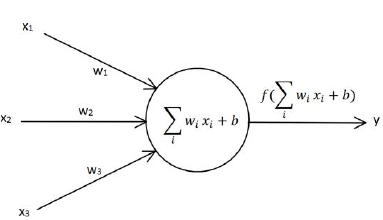如上图，每个神经元都是多输入，单输出的信息处理单元，输入信号通过带权重的连接传递，和阈值对比后得到总输入值，再通过激活函数的处理产生单个输出。 PS.激活函数的意义就是通过进行映射，解决非线性可分问题，激活函数有：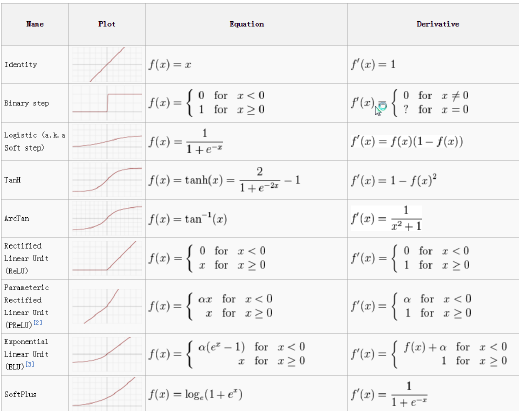其中，常用的激活函数为：sigmoid函数、tanH函数和强大的ReLU函数。 此时，从激活函数到输出有： 输入：

w

1

x

1

+

w

2

x

2

+

x

3

x

3

w_1x_1+w_2x_2+x_3x_3

输出：

O

=

f

(

w

1

x

1

+

w

2

x

2

+

x

3

x

3

+

阈

值

)

O=f(w_1x_1+w_2x_2+x_3x_3+阈值)

为了方便计算，我们常将偏置因子b作为

w

0

w_0

，引入偏置神经元：

x

0

=

−

1

x_0=-1

将阈值也看做加权和的一部分——

w

0

x

0

w_0x_0

（+阈值或-阈值），即引入了

x

0

x_0

列 x0:取值1或-1 w0:阈值 输出：

f

(

w

0

x

0

+

w

1

x

1

+

w

2

x

2

+

.

.

.

+

w

n

x

n

)

f(w_0x_0+w_1x_1+w_2x_2+...+w_nx_n)

和我们刚才所说人脑的神经网络系统一样，我们的人工神经网络也是：输入一定，通过调整权重来影响输出。下面，我们看一看感知器的权值更新计算过程。
感知机权值更新的概念和计算
感知机的权值更新
感知器是怎样更新权值的呢？它通过输入信息的加权汇总，带入激活函数中，计算的输出值与target目标值（原本的标签）进行对比，若有差异，则调整权重，直到满足一定的迭代次数或者达到预设的最小误差值时停止。 简要理解感知器的权值更新过程（具体的推导请参考：《统计学习方法》第二章） 首先，我们要明了，感知机的权值更新同样采用梯度下降的方法： 当感知机采用sigmoid函数作为激活函数时，此时相当于逻辑回归！采用同样的决策边界，可以得到：

O

j

=

{

−

1

,

X

w

<

0

1

,

X

w

>

0

O_j=\begin{cases} -1 ,&Xw<0\\1, &Xw>0\end{cases}

注意：此时采用双极性阈值，取值在-1~1！ i)当

t

=

O

=

s

i

g

n

(

X

w

)

t=O=sign(Xw)

时,无需更新权值； ii)当

t

≠

O

=

s

i

g

n

(

X

w

)

t\not=O=sign(Xw)

时,

{

O

<

0

,

X

w

<

0

,

Δ

w

=

η

(

t

−

O

)

X

=

2

η

X

>

0

，

O

不

断

向

t

=

1

靠

近

O

>

0

,

X

w

>

0

，

Δ

w

=

η

(

t

−

O

)

X

=

−

2

η

X

<

0

，

O

不

断

向

t

=

−

1

靠

近

\begin{cases}O<0,&Xw<0,\Delta w=\eta(t-O)X=2\eta X>0，O不断向t=1靠近\\O>0,&Xw>0，\Delta w=\eta(t-O)X=-2\eta X<0，O不断向t=-1靠近\end{cases}

从而实现权值更新。
感知机详细计算过程
下面，我们用一个例子来说明感知机的计算过程： 某计算节点感知器有3个输入。给定样本：

X

1

=

(

1

,

−

2

)

T

，

X

2

=

(

0

,

1.5

)

T

，

X

3

=

(

−

1

,

1

)

T

X^1=(1,-2)^T，X^2=(0,1.5)^T，X^3=(-1,1)^T

输入向量中需要加入第一个恒等于-1的分量。期望输出向量

d

=

(

−

1

,

−

1

,

1

)

T

d=(-1,-1,1)^T

。初始权向量

w

=

(

0.5

,

1

,

−

1

)

T

w=(0.5,1,-1)^T

,学习率η=0.1，激活函数采用双极性阈值函数。 解：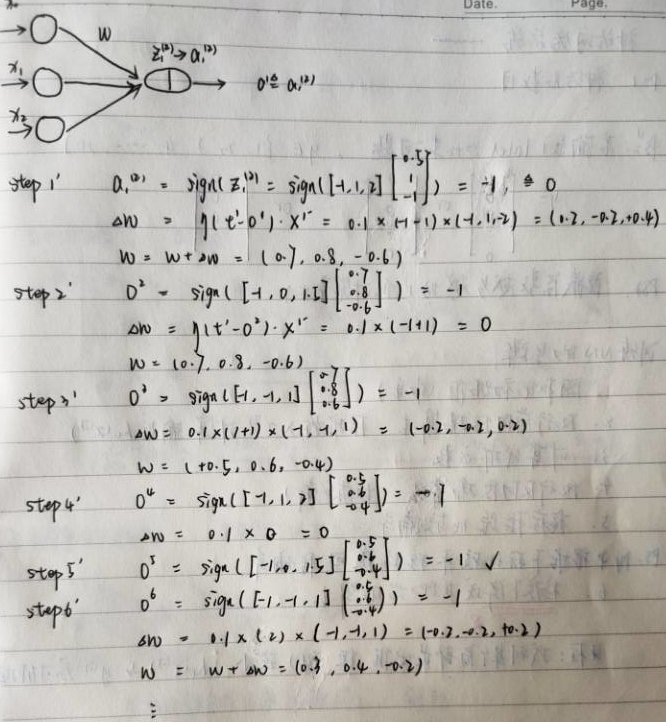增加hidden layer的神经网络——BP神经网络
引入：本文一开始“滴血认亲”的例子就是属于含有一层隐层的神经网络。隐层的甄嬛和皇后这两个“神经元”对输入层信息进行加权汇总并带入激活函数，所得的输出作为输出层的输入。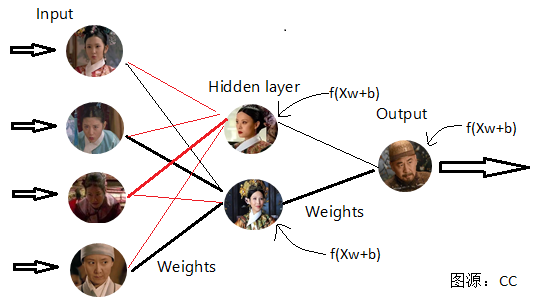(PS.图片为博主原创，转载请注明出处，谢谢！)
为什么要增加隐层？
没有隐层的神经网络可以看做对输入信息的线性组合。单层感知机即是其中一个例子，但是单层感知机的局限是：它只能解决线性可分的“与”，“或”，“非”问题，不能解决线性不可分的"异或"问题。这个时候，增加隐层相当于相当于在第一次sigmoid变换的基础上再次进行sigmoid转换，对数据进行两次映射，可以转换为线性可分问题。可以推广到：通过增加隐层可以解决各种情形的线性不可分问题。有如下图：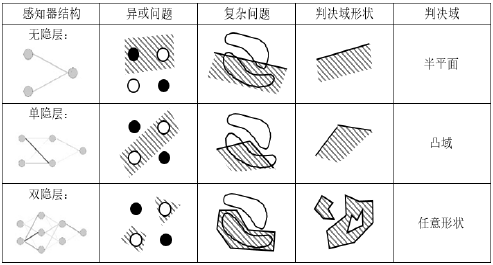PS.关于“或”，“与”，“非”问题大家可以自行百度，或者直接理解为：线性可分问题。

BP(back propagation)神经网络是1986年由Rumelhart和McClelland为首的科学家提出的概念，是一种按照误差逆向传播算法训练的多层前馈神经网络，是目前应用最广泛的神经网络。它的基本思想是梯度下降法，利用梯度搜索技术，以期使网络的实际输出值和期望输出值的误差均方差为最小。

delta学习规则
δ学习规则是一种利用梯度下降法的一般性的学习规则。 首先，BP神经网络的损失函数(Cost Function)：

E

=

1

2

(

t

−

O

)

2

E=\frac{1}{2}(t-O)^2

其中，t为数据原始标签，即为target。O为我们的预测输出。delta学习规则即是通过梯度下降法，使我们的预测输出和目标标签的误差平方和最小。 使用梯度下降法来最小化E的值：

Δ

w

=

−

η

∂

E

∂

w

=

−

η

X

T

(

t

−

O

)

∂

f

(

X

w

)

∂

w

=

η

X

T

δ

,

(

δ

=

−

(

t

−

y

)

∂

f

(

X

w

)

∂

w

)

\Delta w=-\eta \frac{\partial E}{\partial w}=-\eta X^T(t-O)\frac{\partial f(Xw)}{\partial w}=\eta X^T\delta ,(\delta=-(t-y)\frac{\partial f(Xw)}{\partial w})

w

≔

w

+

Δ

w

w≔w+\Delta w

BP神经网络权值更新的梯度下降法推导过程
对于三层网络，推导输出层和隐层权值更新公式。(实质是基于delta规则的链式法则)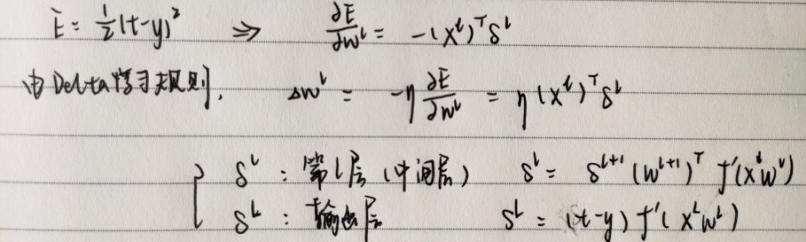具体推导如下： 例：下图为三层神经网络，隐层三节点，使用激活函数为sigmoid函数。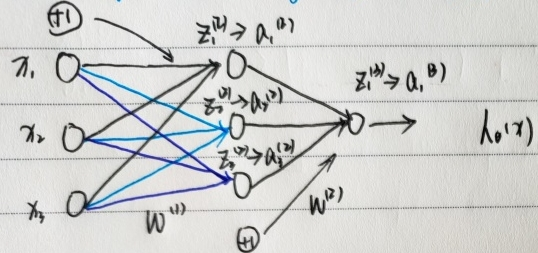PS.在进行后续运算前，对数据向量化处理。输入数据为矩阵：

X

X

，第一层权重为向量：

W

(

1

)

W^{(1)}

,第二层权重为向量：

W

(

2

)

W^{(2)}

,隐层输出矩阵：

Z

(

2

)

Z^{(2)}

,输出层输入矩阵：

a

(

2

)

a^{(2)}

。 已知，

E

=

1

2

(

t

−

O

)

2

E=\frac{1}{2}(t-O)^2

在本例（上图）中可得

E

=

1

2

(

t

−

a

1

(

3

)

)

2

E=\frac{1}{2}(t-a_1^{(3)})^2

S

t

e

p

1

′

Step 1'

输出层—>隐层权值更新：

∂

E

∂

w

(

2

)

=

∂

E

∂

a

1

(

3

)

⋅

∂

a

1

(

3

)

∂

z

1

(

3

)

⋅

∂

z

1

(

3

)

∂

w

(

2

)

=

−

(

t

−

a

1

(

3

)

)

⋅

a

1

(

3

)

(

1

−

a

1

(

3

)

)

⋅

a

(

2

)

\frac{\partial E}{\partial w^{(2)}}=\frac{\partial E}{\partial a_1^{(3)}}·\frac{\partial a_1^{(3)}}{\partial z_1^{(3)}}·\frac{\partial z_1^{(3)}}{\partial w^{(2)}}=-(t-a_1^{(3)})·a_1^{(3)}(1-a_1^{(3)})·a^{(2)}

记:

δ

L

=

−

(

t

−

a

1

(

3

)

)

⋅

a

1

(

3

)

(

1

−

a

1

(

3

)

)

\delta_L=-(t-a_1^{(3)})·a_1^{(3)}(1-a_1^{(3)})

梯度下降更新权值：

w

:

=

w

+

Δ

w

=

w

+

η

⋅

δ

L

⋅

a

(

2

)

w:=w+\Delta w=w+\eta·\delta_L·a^{(2)}

S

t

e

p

2

′

Step2'

隐层—>输入层权值更新：

∂

E

∂

w

(

1

)

=

∂

E

∂

a

1

(

3

)

⋅

∂

a

1

(

3

)

∂

z

1

(

3

)

⋅

∂

z

1

(

3

)

∂

a

(

2

)

⋅

∂

a

(

2

)

∂

z

(

2

)

⋅

∂

z

(

2

)

∂

w

(

1

)

\frac{\partial E}{\partial w^{(1)}}=\frac{\partial E}{\partial a_1^{(3)}}·\frac{\partial a_1^{(3)}}{\partial z_1^{(3)}}·\frac{\partial z_1^{(3)}}{\partial a^{(2)}}·\frac{\partial a^{(2)}}{\partial z^{(2)}}·\frac{\partial z^{(2)}}{\partial w^{(1)}}

=

−

(

t

−

a

1

(

3

)

)

⋅

a

1

(

3

)

(

1

−

a

1

(

3

)

)

⋅

w

(

2

)

⋅

a

(

2

)

(

1

−

a

(

2

)

)

⋅

X

=-(t-a_1^{(3)})·a_1^{(3)}(1-a_1^{(3)})·w^{(2)}·a^{(2)}(1-a^{(2)})·X

=

δ

L

⋅

w

(

2

)

⋅

a

(

2

)

(

1

−

a

(

2

)

)

⋅

X

=\delta_L·w^{(2)}·a^{(2)}(1-a^{(2)})·X

记:

δ

l

=

−

(

t

−

a

1

(

3

)

)

⋅

a

1

(

3

)

(

1

−

a

1

(

3

)

)

⋅

w

(

2

)

⋅

a

(

2

)

(

1

−

a

(

2

)

)

\delta_l=-(t-a_1^{(3)})·a_1^{(3)}(1-a_1^{(3)})·w^{(2)}·a^{(2)}(1-a^{(2)})

梯度下降更新权值：

w

:

=

w

+

Δ

w

=

w

+

η

⋅

δ

L

⋅

w

(

2

)

⋅

a

(

2

)

(

1

−

a

(

2

)

)

⋅

X

w:=w+\Delta w=w+\eta·\delta_L·w^{(2)}·a^{(2)}(1-a^{(2)})·X

BP神经网络计算实例
基本BP算法包括信号的前向传播和误差的反向传播两个过程,我们通过一个例子详细计算BP神经网络的信号前向传播、误差反向反馈过程。 以上图为例，

S

t

e

p

1

′

信

号

向

前

传

播

Step 1' 信号向前传播

第一层——数据输入为：

X

1

,

X

2

,

X

3

即

a

1

(

1

)

，

a

2

(

1

)

，

a

3

(

1

)

X_1,X_2,X_3即a_1^{(1)}，a_2^{(1)}，a_3^{(1)}

中间层——

a

1

(

2

)

=

g

(

z

1

(

2

)

)

，

z

1

(

2

)

=

w

01

(

1

)

x

0

+

w

11

(

1

)

x

1

+

w

21

(

1

)

x

2

+

w

31

(

1

)

x

3

a_1^{(2)}=g(z_1^{(2)} )，z_1^{(2)}=w_{01}^{(1)} x_0+w_{11}^{(1)} x_1+w_{21}^{(1)} x_2+w_{31}^{(1)} x_3

a

2

(

2

)

=

g

(

z

2

(

2

)

)

，

z

2

(

2

)

=

w

02

(

1

)

x

0

+

w

12

(

1

)

x

1

+

w

22

(

1

)

x

2

+

w

32

(

1

)

x

3

a_2^{(2)}=g(z_2^{(2)} )，z_2^{(2)}=w_{02}^{(1)} x_0+w_{12}^{(1)} x_1+w_{22}^{(1)} x_2+w_{32}^{(1)} x_3

a

3

(

2

)

=

g

(

z

3

(

2

)

)

，

z

3

(

2

)

=

w

03

(

1

)

x

0

+

w

13

(

1

)

x

1

+

w

23

(

1

)

x

2

+

w

33

(

1

)

x

3

a_3^{(2)}=g(z_3^{(2)} )，z_3^{(2)}=w_{03}^{(1)} x_0+w_{13}^{(1)} x_1+w_{23}^{(1)} x_2+w_{33}^{(1)} x_3

输出层——

O

=

h

w

(

x

)

=

a

1

(

3

)

=

g

(

z

1

(

3

)

)

,

z

1

(

3

)

=

w

01

(

2

)

a

0

(

2

)

+

w

11

(

2

)

a

1

(

2

)

+

w

21

(

2

)

a

2

(

2

)

+

w

31

(

2

)

a

3

(

2

)

O=h_w (x)=a_1^{(3)}=g(z_1^{(3)}),z_1^{(3)}=w_{01}^{(2)}a_0^{(2)}+w_{11}^{(2)}a_1^{(2)}+w_{21}^{(2)}a_2^{(2)}+w_{31}^{(2)}a_3^{(2)}

S

t

e

p

2

′

误

差

反

向

反

馈

Step 2'误差反向反馈

输出层—>隐层

δ

L

=

−

(

t

−

a

1

(

3

)

)

⋅

a

1

(

3

)

(

1

−

a

1

(

3

)

)

\delta_L=-(t-a_1^{(3)})·a_1^{(3)}(1-a_1^{(3)})

隐层—>输入层

δ

l

=

δ

L

⋅

w

(

2

)

⋅

a

(

2

)

(

1

−

a

(

2

)

)

\delta_l=\delta_L·w^{(2)}·a^{(2)}(1-a^{(2)})

S

t

e

p

3

′

权

值

更

新

Step 3'权值更新

W

(

2

)

:

=

W

(

2

)

+

η

⋅

δ

L

⋅

a

(

2

)

W^{(2)}:=W^{(2)}+\eta·\delta_L·a^{(2)}

W

(

1

)

:

=

W

(

1

)

+

η

⋅

δ

l

⋅

X

W^{(1)}:=W^{(1)}+\eta·\delta_l·X

notes：感知机和线性回归、逻辑回归的关联
① 感知机+sigmoid/logit函数——>逻辑回归 ② 感知机+f(x)=wx+b线性函数——>线性回归 ③ 三层网络+sigmoid/logit函数——>非线性回归
总结
本文主要介绍了神经网络入门必须要了解的两个算法：感知机和BP神经网络。从人工神经网络的由来到感知机算法的原理、BP算法的原理及推导。
python实现——sklearn葡萄酒分类
导入所需库
from sklearn.neural_network import MLPClassifier
from sklearn.model_selection import train_test_split #用于数据分割
from sklearn.preprocessing import StandardScaler #做数据标准化
from sklearn.metrics import classification_report,confusion_matrix
import numpy as np

导入所需数据
data = np.genfromtxt('data/wine_data.csv', delimiter=',')
data

数据转化——区分X和Y
x_data = data[:,1:]
y_data = data[:,0]   #第一列是标签值

数据分割
x_train, x_test, y_train, y_test = train_test_split(x_data, y_data)

数据标准化
scaler = StandardScaler()
x_train = scaler.fit_transform(x_train)
x_test = scaler.fit_transform(x_test)

调用神经网络包并传入参数，拟合模型
mlp = MLPClassifier(hidden_layer_sizes=(100, 50),max_iter=500)
mlp.fit(x_train, y_train)

模型预测并输出混淆矩阵
predictions = mlp.predict(x_test)
print(classification_report(y_test, predictions))
print(confusion_matrix(y_test,predictions))

展开全文神经网络 权值更新 机器学习
• 我们以单隐层感知器为例进行BP网络模型的说明，一般习惯将单隐层感知器称为三层感知器，所谓三层包括了输入层，隐层输出层。 三层感知器中，输入向量为X=(x1,x2,⋯&ThinSpace;,xi,⋯&ThinSpace;,xn)T\bm X...
郑重声明：以下内容，完全参考韩力群编著的《人工神经网络理论，设计及应用》

BP算法的基本思想是，学习过程由信号的正向传播与误差的反向传播两个过程组成。

1. BP网络模型
我们以单隐层感知器为例进行BP网络模型的说明，一般习惯将单隐层感知器称为三层感知器，所谓三层包括了输入层，隐层和输出层。 三层感知器中，输入向量为

X

=

(

x

1

,

x

2

,

⋯

,

x

i

,

⋯

,

x

n

)

T

\bm X=(x_1,x_2,\cdots,x_i,\cdots,x_n)^T

，图中

x

0

=

−

1

x_0=-1

是为隐层神经元引入阈值而设置的；隐层输出向量为

Y

=

(

y

1

,

y

2

,

⋯

,

y

j

,

⋯

,

y

m

)

T

\bm Y=(y_1,y_2,\cdots,y_j,\cdots,y_m)^T

，图中

y

0

=

−

1

y_0=-1

是为输出层神经元引入阈值而设置的；输出层的向量为

O

=

(

o

1

,

o

2

,

⋯

,

o

k

,

⋯

,

o

l

)

T

\bm O=(o_1,o_2,\cdots,o_k,\cdots,o_l)^T

；期望输出向量为

d

=

(

d

1

,

d

2

,

⋯

,

d

k

,

⋯

,

d

l

)

T

\bm d=(d_1,d_2,\cdots,d_k,\cdots,d_l)^T

。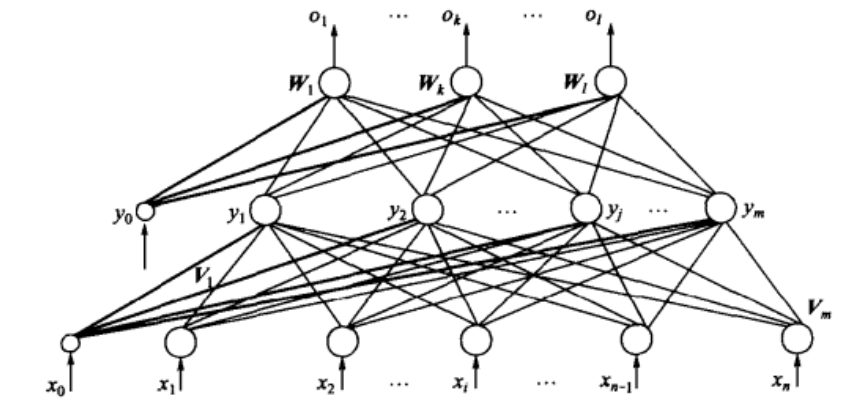输入层到隐层之间的权值矩阵用

V

\bm V

表示，

V

=

(

V

1

,

V

2

,

⋯

,

V

j

,

⋯

,

V

m

)

\bm V=(\bm V_1,\bm V_2,\cdots,\bm V_j,\cdots,\bm V_m)

，其中列向量

V

j

\bm V_j

为隐层第

j

j

个神经元对应的权向量，隐层到输出层之间的权值矩阵用

W

\bm W

表示，

W

=

(

W

1

,

W

2

,

⋯

,

W

k

,

⋯

,

W

l

)

\bm W=(\bm W_1,\bm W_2,\cdots,\bm W_k,\cdots,\bm W_l)

，其中列向量

W

k

\bm W_k

为输出层第

k

k

个神经元对应的权向量。 下面分析各层信号之间的数学关系。
对于输出层，有：

o

k

=

f

(

n

e

t

k

)

k

=

1

,

2

,

⋯

,

l

(1.1)

n

e

t

k

=

∑

j

=

0

m

w

j

k

y

j

k

=

1

,

2

,

⋯

,

l

(1.2)

对于隐层，有：

y

i

=

f

(

n

e

t

j

)

j

=

1

,

2

,

⋯

,

m

(1.3)

n

e

t

j

=

∑

i

=

0

n

v

i

j

x

i

j

=

1

,

2

,

⋯

,

m

(1.4)

以上两式中，激活函数（转移函数）

f

(

x

)

f(x)

均为单极性Sigmoid函数：

f

(

x

)

=

1

1

+

e

−

x

(1.5)

f(x)=\frac{1}{1+{\rm e}^{-x}}\tag{1.5}

f

(

x

)

f(x)

具有连续、可导的特点，且有：

f

′

(

x

)

=

f

(

x

)

(

1

−

f

(

x

)

)

(1.6)

f'(x)=f(x)(1-f(x))\tag{1.6}

根据需要，也可以采用双极性Sigmoid函数（或称双曲线正切函数）：

f

(

x

)

=

1

−

e

−

x

1

+

e

−

x

(1.7)

f(x)=\frac{1-{\rm e}^{-x}}{1+{\rm e}^{-x}}\tag{1.7}

式(1.1) ~ (1.5)共同构成了三层感知器的数学模型。
2. BP学习算法
下面一三层感知器为例介绍BP学习算法，然后将所得结论推广到一般多层感知器的情况。
2.1 网络误差定义和权值调整思路
在进行下面推导的过程中，我们必须要重视以下符号的含义:

w

j

k

h

w_{jk}^h

：表示第

h

−

1

h-1

隐层第

j

j

个神经元的输出值 传递到第

h

h

隐层第

k

k

个神经元时的缩放比例，称之为权值。

y

j

h

y^h_j

：表示第

h

h

隐层第

j

j

个神经元的输出值，也是

h

+

1

h+1

层神经元d 输入值经过权重

w

j

k

h

+

1

w_{jk}^{h+1}

缩放后的一部分。

n

e

t

j

h

{\rm net}_j^h

：表示第

h

h

隐层第

j

j

个神经元的集结输入值，

n

e

t

j

h

=

∑

j

=

0

m

w

i

j

h

y

i

h

−

1

{\rm net}_j^h=\sum^m_{j=0}w^h_{ij}y^{h-1}_i

。

f

(

n

e

t

j

h

)

f({\rm net}_j^h)

：表示第

h

h

隐层第

j

j

个神经元的激活函数（转移函数）,即：

y

j

h

=

f

(

n

e

t

j

h

)

y_j^h=f({\rm net}_j^h)

δ

j

h

\delta^h_j

：表示第

h

h

隐层第

j

j

个神经元的输出误差信号，也称为学习信号，这是因为网络误差函数

E

E

对第

h

h

隐层第

j

j

个神经元的

n

e

t

j

h

{\rm net}_j^h

求偏导、并取反，即：

δ

j

h

=

−

∂

E

n

e

t

j

h

=

−

∂

E

∂

y

j

h

∂

y

j

h

∂

n

e

t

j

h

=

−

∂

E

∂

y

j

h

f

′

(

n

e

t

j

h

)

\delta^h_j=-\frac{\partial E}{{\rm net}_j^h}=-\frac{\partial E}{\partial y^h_j}\frac{\partial y^h_j}{\partial {\rm net}^h_j}=-\frac{\partial E}{\partial y^h_j}f'({\rm net}^h_j)

Δ

w

i

j

h

\Delta w^h_{ij}

：表示经过一些列计算，确定当前第

h

−

1

h-1

隐层第

i

i

个神经元到第

h

h

隐层第

j

j

个神经元的权值要调整的具体数值

Δ

w

i

j

h

=

η

δ

j

h

y

i

h

−

1

\Delta w^h_{ij}=\eta\delta^h_jy^{h-1}_i

理解BP算法的核心是要懂得，所有的推导铺垫都是在为某一隐层或输出层的权值函数

v

i

j

v_{ij}

或

w

j

k

w_{jk}

做铺垫的。如果把某一隐层或输出层的权值数看作是矩阵，则应该是这样的：

V

(

n

+

1

)

×

m

=

(

V

1

,

V

2

,

⋯

,

V

m

)

=

(

v

11

v

12

⋯

v

1

m

v

21

v

22

⋯

v

2

m

⋮

⋱

⋮

v

(

n

+

1

)

1

v

(

n

+

1

)

2

⋯

v

(

n

+

1

)

m

)

V^{(n+1)\times m}=(V_1,V_2,\cdots,V_m)=\begin{pmatrix}v_{11}&v_{12}&\cdots&v_{1m}\\ v_{21}&v_{22}&\cdots&v_{2m}\\ \vdots& &\ddots&\vdots\\ v_{(n+1)1}&v_{(n+1)2}&\cdots&v_{(n+1)m} \end{pmatrix}

理解这个矩阵构造的关键在于理解：
列数代表当前隐藏层的神经元个数，当前矩阵显示本隐藏层有

m

m

个神经元；当前隐藏层的权值矩阵的每一列代表相应的一个神经元所对应于上一层隐藏层(或输入层)神经元的一系列权值，例如上式中

V

1

V_1

表示该隐藏层第一个神经元对应于上一隐藏层各神经元的权值，假设上一层有

n

n

个神经元，由于还要考虑将阈值引入到当前隐藏层中，故上一层隐藏层还要加一个输出值固定为"-1"的神经元，因此上一层共有

n

+

1

n+1

个神经元,因此

V

1

=

(

v

11

,

v

21

,

v

31

,

⋯

,

v

(

n

+

1

)

1

)

T

V_1=(v_{11},v_{21},v_{31},\cdots,v_{(n+1)1})^T

—————————————————————————————————————————— 还要理解好学习信号

δ

j

h

\delta^h_{j}

，他表示第

h

h

个隐藏层中第

j

j

个神经元的学习信号，注意，

δ

j

h

\delta^h_{j}

是标量，但他将负责参与求出权值

V

j

V_j

列向量的一系列列权值，因为

δ

j

h

=

−

∂

E

∂

n

e

t

j

h

=

−

∂

E

∂

y

j

h

f

′

(

n

e

t

j

h

)

\delta^h_{j}=-\frac{\partial E}{\partial net^h_j}=-\frac{\partial E}{\partial y^h_j}f'(net^h_j)

y

j

h

=

f

(

n

e

t

j

h

)

=

f

(

∑

i

=

0

n

y

h

−

1

v

i

j

)

y^h_j=f(net^h_j)=f\left(\sum^n_{i=0}y^{h-1}v_{ij}\right)

所以权值

Δ

V

j

h

=

Δ

v

i

j

h

=

η

δ

j

h

y

i

h

−

1

i

=

0

,

1

,

2

,

⋯

,

n

对于同一条训练数据而言，不同的神经元有不同的

δ

j

h

\delta^h_j

；对于同一层的同一位置的神经元，不同的训练数据有不同的

δ

j

h

\delta^h_j

。

以上说明中多写了一部分结论，具体推导请看下文。

网络误差定义 当网络输出与期望输出不等时，存在输出误差

E

E

，定义如下：

E

=

1

2

∣

∣

d

−

O

∣

∣

2

2

=

1

2

∑

k

=

1

l

(

d

k

−

o

k

)

2

(2.1)

E=\frac{1}{2}||\bm d-\bm O||_2^2=\frac{1}{2}\sum^l_{k=1}(d_k-o_k)^2\tag{2.1}

将以上误差定义是展开至隐层，有：

E

=

1

2

∑

k

=

1

l

[

d

k

−

f

(

n

e

t

k

)

]

2

=

1

2

∑

k

=

1

l

[

d

k

−

f

(

∑

j

=

0

m

w

j

k

y

j

)

]

2

(2.2)

\begin{aligned}E&=\frac{1}{2}\sum^l_{k=1}[d_k-f({\rm net}_k)]^2\\ &=\frac{1}{2}\sum^l_{k=1}[d_k-f(\sum^m_{j=0}w_{jk}y_j)]^2 \tag{2.2}\end{aligned}

进一步展开至输入层，有：

E

=

1

2

∑

k

=

1

l

{

d

k

−

f

[

∑

j

=

0

m

w

j

k

f

(

n

e

t

j

)

]

}

2

=

1

2

∑

k

=

1

l

{

d

k

−

f

[

∑

j

=

0

m

w

j

k

f

(

∑

i

=

0

n

v

i

j

x

i

)

]

}

2

(2.3)

\begin{aligned}E&=\frac{1}{2}\sum^l_{k=1}\{d_k-f[\sum^m_{j=0}w_{jk}f({\rm net}_j)]\}^2\\ &=\frac{1}{2}\sum^l_{k=1}\{d_k-f[\sum^m_{j=0}w_{jk}f(\sum^n_{i=0}v_{ij}x_i)]\}^2 \tag{2.3}\end{aligned}

权值调整思路 从上式可以看出，网络误差是关于各层权值

w

j

k

、

v

i

j

w_{jk}、v_{ij}

的函数，因此调整权值可以改变误差

E

E

。 调整权值的原则是使误差不断地减小，因此，应使权值的调整量与误差的梯度下降成正比，即：

Δ

w

j

k

=

−

η

∂

E

∂

w

j

k

j

=

0

,

1

,

2

,

⋯

,

m

;

k

=

1

,

2

,

⋯

,

l

(2.4a)

Δ

v

i

j

=

−

η

∂

E

∂

v

i

j

i

=

0

,

1

,

2

,

⋯

,

n

;

j

=

1

,

2

,

⋯

,

m

(2.4b)

式中，符号表示梯度下降，常数

η

∈

(

0

,

1

)

\eta\in(0,1)

表示比例系数，在训练中反映学习速率。
2.2 BP算法的推导
式

(

2.4

)

(2.4)

仅是对权值调整思路的数学表达式，而不是具体的权值调整计算式。下面推导三层BP算法权值调整的计算式。事先约定，在全部推导过程中，对输出层均有

j

=

0

,

1

,

2

,

⋯

,

m

j=0,1,2,\cdots,m

，

k

=

1

,

2

,

⋯

,

l

k=1,2,\cdots,l

；对隐层均有

i

=

0

,

1

,

2

,

⋯

,

n

i=0,1,2,\cdots,n

，

j

=

1

,

2

,

⋯

,

m

j=1,2,\cdots,m

。这是因为如果把当前隐层当成是相对于下一层的输入层，则必须要夹带

y

0

=

−

1

y_0=-1

的一个当前层神经元作为引入下一层的阈值的设置；而如果仅仅是求取当前隐层的输出值，则无须考虑“为下一隐层引入阈值而设置的当前隐层中的

y

0

=

−

1

y_0=-1

”，因为作为下一层的输入值，他永远是等于“-1”。
对于输出层，式

(

2.4

a

)

(2.4a)

可写为：

Δ

w

j

k

=

−

η

∂

E

∂

w

j

k

=

−

η

∂

E

∂

n

e

t

k

∂

n

e

t

k

∂

w

j

k

(2.5a)

\Delta w_{jk}=-\eta\frac{\partial E}{\partial w_{jk}}=-\eta\frac{\partial E}{\partial {\rm net}_k}\frac{\partial {\rm net}_k}{\partial w_{jk}}\tag{2.5a}

对于隐层，式

(

2.4

b

)

(2.4b)

可写为：

Δ

v

i

j

=

−

η

∂

E

∂

v

i

j

=

−

η

∂

E

∂

n

e

t

j

∂

n

e

t

j

∂

v

i

j

(2.5b)

\Delta v_{ij}=-\eta\frac{\partial E}{\partial v_{ij}}=-\eta\frac{\partial E}{\partial {\rm net}_j}\frac{\partial {\rm net}_j}{\partial v_{ij}}\tag{2.5b}

对于输出层和隐层各定义一个误差信号，令：

δ

k

o

=

−

∂

E

∂

n

e

t

k

(2.6a)

\delta^o_k=-\frac{\partial E}{\partial {\rm net}_k}\tag{2.6a}

δ

j

y

=

−

∂

E

∂

n

e

t

j

(2.6b)

\delta^y_j=-\frac{\partial E}{\partial {\rm net}_j}\tag{2.6b}

综合应用式

(

1.2

)

(1.2)

和式

(

2.6

a

)

(2.6a)

，可将式

(

2.5

a

)

(2.5a)

的权值调整式改写为：

Δ

w

j

k

=

η

δ

k

o

y

j

(2.7a)

\Delta w_{jk}=\eta\delta^o_ky_j\tag{2.7a}

Δ

v

i

j

=

η

δ

j

y

x

i

(2.7b)

\Delta v_{ij}=\eta\delta^y_jx_i\tag{2.7b}

可以看出，只要计算出式

(

2.7

)

(2.7)

中的误差信号

δ

k

o

\delta^o_k

和

δ

j

y

\delta^y_j

，权值调整量的计算推导即可完成。
对于输出层，

δ

k

o

\delta^o_k

可展开为：

δ

k

o

=

−

∂

E

∂

n

e

t

k

=

−

∂

E

∂

o

k

∂

o

k

∂

n

e

t

k

=

−

∂

E

∂

o

k

f

′

(

n

e

t

k

)

(2.8a)

\delta^o_k=-\frac{\partial E}{\partial {\rm net}_k}=-\frac{\partial E}{\partial o_k}\frac{\partial o_k}{\partial {\rm net}_k}=-\frac{\partial E}{\partial o_k}f'({\rm net}_k)\tag{2.8a}

对于隐层，

δ

j

y

\delta^y_j

可展开为：

δ

j

y

=

−

∂

E

∂

n

e

t

j

=

−

∂

E

∂

y

j

∂

y

j

∂

n

e

t

j

=

−

∂

E

∂

y

j

f

′

(

n

e

t

j

)

(2.8b)

\delta^y_j=-\frac{\partial E}{\partial {\rm net}_j}=-\frac{\partial E}{\partial y_j}\frac{\partial y_j}{\partial {\rm net}_j}=-\frac{\partial E}{\partial y_j}f'({\rm net}_j)\tag{2.8b}

下面求式

(

2.8

)

(2.8)

中网络误差

E

E

对各层输出的偏导. 对于输出层，利用式

(

2.1

)

(2.1)

，可得：

∂

E

∂

o

k

=

−

(

d

k

−

o

k

)

(2.9a)

\frac{\partial E}{\partial o_k}=-(d_k-o_k)\tag{2.9a}

对于隐层，利用式

(

2.2

)

(2.2)

，可得：

∂

E

∂

y

j

=

−

∑

k

=

1

l

(

d

k

−

o

k

)

f

′

(

n

e

t

k

)

w

j

k

(2.9b)

\frac{\partial E}{\partial y_j}=-\sum^l_{k=1}(d_k-o_k)f'({\rm net}_k)w_{jk}\tag{2.9b}

利用以上结果代入式

(

2.8

)

(2.8)

，如果激活函数全部利用单极性Sigmoid函数，即式

(

1.6

)

(1.6)

，可得：

δ

k

o

=

(

d

k

−

o

k

)

o

k

(

1

−

o

k

)

(2.10a)

\delta^o_k=(d_k-o_k)o_k(1-o_k)\tag{2.10a}

δ

j

y

=

−

[

∑

k

=

1

l

(

d

k

−

o

k

)

f

′

(

n

e

t

k

)

w

j

k

]

f

′

(

n

e

t

j

)

=

(

∑

k

=

1

l

δ

k

o

w

j

k

)

y

j

(

1

−

y

j

)

(2.10b)

\begin{aligned} \delta^y_j&=-\left[\sum^l_{k=1}(d_k-o_k)f'({\rm net}_k)w_{jk}\right]f'({\rm net}_j)\\ &=\left(\sum^l_{k=1}\delta^o_kw_{jk}\right)y_j(1-y_j)\tag{2.10b} \end{aligned}

至此，两个误差信号已经推到完成，将式

(

2.10

)

(2.10)

带回到式

(

2.7

)

(2.7)

，便得到了三层感知器的利用单隐层Sigmoid函数作激活函数的BP学习算法权值调整计算公式，即

{

Δ

w

j

k

=

η

δ

k

o

y

j

=

η

(

d

k

−

o

k

)

o

k

(

1

−

o

k

)

y

j

Δ

v

i

j

=

η

δ

j

y

x

i

=

η

(

∑

k

=

1

l

δ

k

o

w

j

k

)

y

j

(

1

−

y

j

)

x

i

(2.11)

\left\{\begin{matrix}\Delta w_{jk}=\eta\delta^o_ky_j=\eta(d_k-o_k)o_k(1-o_k)y_j\tag{2.11}\\ \Delta v_{ij}=\eta\delta^y_jx_i=\eta\left(\sum^l_{k=1}\delta^o_kw_{jk}\right)y_j(1-y_j)x_i \end{matrix}\right.

对于一般的多层感知器，设共有

h

h

个隐层，按前向顺序各隐层神经元数分别记为

m

1

,

m

2

,

⋯

,

m

h

m_1,m_2,\cdots,m_h

，各隐层输出分别记为

y

1

,

y

2

,

⋯

,

y

h

y^1,y^2,\cdots,y^h

，各层权值矩阵分别记为

W

1

,

W

2

,

⋯

,

W

h

,

W

h

+

1

\bm W^1,\bm W^2,\cdots,\bm W^h,\bm W^{h+1}

，则各层的权值调整计算公式为：
输出层

Δ

w

j

k

h

+

1

=

η

δ

k

o

y

j

h

j

=

0

,

1

,

2

,

⋯

,

m

h

;

k

=

1

,

2

,

⋯

,

l

第

h

h

隐层

Δ

w

i

j

h

=

η

δ

j

h

y

i

h

−

1

=

η

(

∑

k

=

1

l

δ

k

o

w

j

k

h

+

1

)

f

′

(

n

e

t

j

h

)

y

i

h

−

1

i

=

0

,

1

,

2

,

⋯

,

m

h

−

1

；

j

=

1

,

2

,

⋯

,

m

h

按以上规律逐层类推，则第一隐层权值调整计算公式：

Δ

w

p

q

1

=

η

δ

q

1

x

p

=

η

(

∑

r

=

1

m

2

δ

r

2

w

q

r

2

)

f

′

(

n

e

t

q

1

)

x

p

p

=

0

,

1

,

2

,

⋯

,

n

；

q

=

1

,

2

,

⋯

,

m

1

三层感知器的BP学习算法也可以写成向量形式。 对于输出层而言，设最后一隐层的输出

Y

=

(

y

0

,

y

1

,

y

2

,

⋯

,

y

i

,

⋯

,

y

m

)

T

，

δ

o

=

(

δ

1

o

,

δ

2

o

,

⋯

,

δ

k

o

,

⋯

,

δ

l

o

)

T

\bm Y=(y_0,y_1,y_2,\cdots,y_i,\cdots,y_m)^T，\bm \delta^o=(\delta^o_1,\delta^o_2,\cdots,\delta^o_k,\cdots,\delta^o_l)^T

，则：

Δ

W

=

η

(

Y

(

δ

o

)

T

)

(2.12a)

\Delta \bm W=\eta(\bm Y (\bm\delta^o)^T)\tag{2.12a}

对于隐层而言，设输入层的输入

X

=

(

x

0

,

x

1

,

x

2

,

⋯

,

x

i

,

⋯

,

x

n

)

T

，

δ

y

=

(

δ

1

y

,

δ

2

y

,

⋯

,

δ

j

y

,

⋯

,

δ

m

y

)

T

\bm X=(x_0,x_1,x_2,\cdots,x_i,\cdots,x_n)^T，\bm\delta^y=(\delta^y_1,\delta^y_2,\cdots,\delta^y_j,\cdots,\delta^y_m)^T

，则：

Δ

V

=

η

(

X

(

δ

y

)

T

)

\Delta\bm V=\eta(\bm X(\bm\delta^y)^T)

容易看出，在BP学习算法中，各层权值调整公式形式上是一样的，均有3各因素决定，即：学习率

η

\eta

、本层输出的误差信号

δ

\bm\delta

以及本层输入信号

Y

(

或

X

)

\bm Y(或\bm X)

。
展开全文• 感知机BP神经网络 感知器作为人工神经网络中最基本的单元，有多个输入一个输出组成。虽然我们的目的是学习很多神经单元互连的网络，但是我们还是需要先对单个的神经单元进行研究。 感知机模型 感知机(perceptron...
感知机与BP神经网络
感知器作为人工神经网络中最基本的单元，有多个输入和一个输出组成。虽然我们的目的是学习很多神经单元互连的网络，但是我们还是需要先对单个的神经单元进行研究。
感知机模型
感知机(perceptron)是二类分类的线性分类模型，其输入为实例的特征向量，输出为实例的类别（取+1和-1）。
感知器定义
假设输入空间(特征向量)是 χ⊆Rn,输出空间为Y={+1,−1}，输入x⊆χ表示实例的特征向量，对应于输入空间的点;输出 y⊆Y表示实例的类别，则由输入空间到输出空间的表达形式为： f(x)=sign(w∗x+b) 称为感知机，其中w叫作权值，b叫作偏置。sign是符号函数，即： sign(x)={−1 x<0 ，-1 x≥0}
感知器的网络模型的拓扑结构图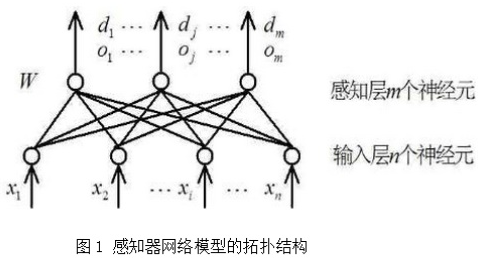感知器算法主要流程
首先得到n个输入，再将每个输入值加权，然后判断感知器输入的加权和最否达到某一阀值v，若达到，则通过sign函数输出1，否则输出-1。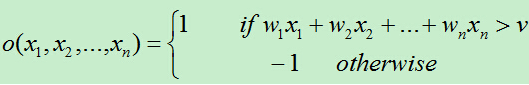为了统一表达式，我们将上面的阀值v设为-w0，新增变量x0=1,这样就可以使用w0x0+w1x1+w2x2+…+wnxn>0来代替上面的w1x1+w2x2+…+wnxn>v。于是有：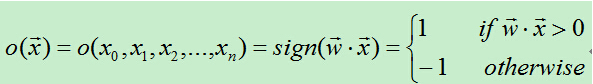从上面的公式可知，当权值向量确定时，就可以利用感知器来做分类。
感知器的权值
训练集线性可分时 --> 感知器训练法则 为了得到可接受的权值，通常从随机的权值开始，然后利用训练集反复训练权值，最后得到能够正确分类所有样例的权向量。
1 初始化权向量w=(w0,w1,…,wn)，将权向量的每个值赋一个随机值。2 对于每个训练样例，首先计算其预测输出：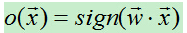3 当预测值不等于真实值时则利用如下公式修改权向量：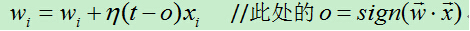各符号含义：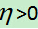代表学习速率，t代表样例的目标输出，o代表感知器输出。4 重复2和3，直到训练集中没有被错分的样例。
训练集线性不可分时 --> delta法则（又叫增量法则， LMS法则，Adaline法则，Windrow-Hoff法则） 由于在真实情况下，并不能保证训练集是线性可分的。因而，当训练集线性不可分时该如何训练感知器呢？这时我们使用delta法则，通过这种方式可以找出收敛到目标的最佳近似值，我们将训练误差函数定义为：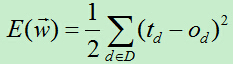其中D：训练集合，td为目标输出，od为感知器输出. 随机梯度下降算法过程如下：
初始化权向量w，将权向量的每个值取一个随机值。对每个训练样例，分别执行以下操作： 　　* 通过感知器得到样例的输出o。 　　* 根据感知器的输出，修改权向量w。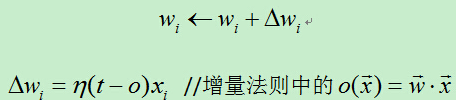重复第2步，当训练样例的误差率小于设定的阀值时，算法终止。 算法条件：误差损失函数需要对权向量可微；假设空间包含连续参数化的假设。 可能存在的问题：若误差曲面有多个局部极小值，则不能保证达到全局最优。
梯度下降法则的推导
梯度下降算法的核心就是每次向损失函数下降最陡峭的方向移动，而最陡峭的方向通常就是损失函数对权向量求偏导数得到的向量的反方向。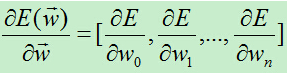逐个计算每个分量每次的权重更新量为：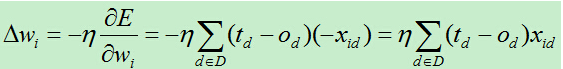此方法将所有训练集权值计算了一个总和，然后将权值更新。此方法更新依次权值需要将所有训练集全部训练一次，故而速度较慢，效率较低。因为速度慢所以有了随机梯度下降算法，权向量更新公式为：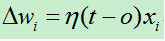它在每个样例中迭代的过程中都会进行权值更新，通过这种方式能更加灵活的调整权值，使得权值以更快的速度收敛。
神经网络
定义
简单来说就是将单个感知器作为一个神经网络节点，然后用此类节点组成一个层次网络结构，我们称此网络即为人工神经网络。当网络的层次大于等于3层（输入层+隐藏层（大于等于1）+输出层）时，我们称之为多层人工神经网络。
神经元的选择
由于sign函数时非连续函数，这使得它不可微，因而不能使用上面的梯度下降算法来最小化损失函数。增量法每个输出都是输入的线性组合，这样当多个线性单元连接在一起后最终也只能得到输入的线性组合，这和只有一个感知器单元节点没有很大不同。 sigmoid函数（Logistic函数）。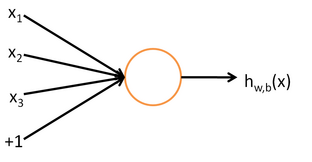公式：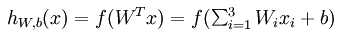其中，该单元也可以被称作是Logistic回归模型。当将多个单元组合起来并具有分层结构时，就形成了神经网络模型。下图展示了一个具有一个隐含层的神经网络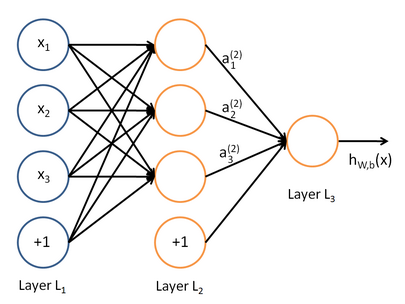公式：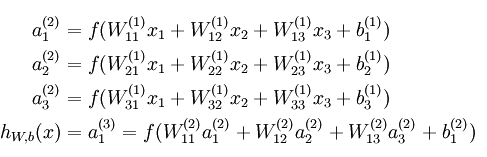同理，可以拓展到2,3…个隐含层
感知器与BP神经网络代码实现
% 第一章  感知器
% 1. 感知器神经网络的构建
% 1.1  生成网络
net=newp([0 2],1);%单输入，输入值为[0,2]之间的数
inputweights=net.inputweights{1,1};%第一层的权重为1
biases=net.biases{1};%阈值为1
% 1.2  网络仿真

net=newp([-2 2;-2 2],1);%两个输入，一个神经元，默认二值激活
net.IW{1,1}=[-1 1];%权重，net.IW{i,j}表示第i层网络第j个神经元的权重向量
net.IW{1,1}
net.b{1}=1;
net.b{1}
p1=[1;1],a1=sim(net,p1)
p2=[1;-1],a2=sim(net,p2)
p3={[1;1] [1 ;-1]},a3=sim(net,p3) %两组数据放一起
p4=[1 1;1 -1],a4=sim(net,p4)%也可以放在矩阵里面
net.IW{1,1}=[3,4];
net.b{1}=;
a1=sim(net,p1)
% 1.3  网络初始化
net=init(net);
wts=net.IW{1,1}
bias=net.b{1}
% 改变权值和阈值为随机数
net.inputweights{1,1}.initFcn='rands';
net.biases{1}.initFcn='rands';
net=init(net);
bias=net.b{1}
wts=net.IW{1,1}
a1=sim(net,p1)

% 2. 感知器神经网络的学习和训练
% 1 网络学习
net=newp([-2 2;-2 2],1);
net.b{1}=;
w=[1 -0.8]
net.IW{1,1}=w;
p=[1;2];
t=;
a=sim(net,p)
e=t-a
help learnp
dw=learnp(w,p,[],[],[],[],e,[],[],[],[],[])
w=w+dw
net.IW{1,1}=w;
a=sim(net,p)

net = newp([0 1; -2 2],1);
P = [0 0 1 1; 0 1 0 1];
T = [0 1 1 1];
Y = sim(net,P)
net.trainParam.epochs = 20;
net = train(net,P,T);
Y = sim(net,P)

% 2 网络训练
net=init(net);
p1=[2;2];t1=0;p2=[1;-2];t2=1;p3=[-2;2];t3=0;p4=[-1;1];t4=1;
net.trainParam.epochs=1;
net=train(net,p1,t1)
w=net.IW{1,1}
b=net.b{1}
a=sim(net,p1)
net=init(net);
p=[[2;2] [1;-2] [-2;2] [-1;1]];
t=[0 1 0 1];
net.trainParam.epochs=1;
net=train(net,p,t);
a=sim(net,p)
net=init(net);
net.trainParam.epochs=2;
net=train(net,p,t);
a=sim(net,p)
net=init(net);
net.trainParam.epochs=20;
net=train(net,p,t);
a=sim(net,p)

% 3. 二输入感知器分类可视化问题
P=[-0.5 1 0.5 -0.1;-0.5 1 -0.5 1];
T=[1 1 0 1]
net=newp([-1 1;-1 1],1);
plotpv(P,T);
plotpc(net.IW{1,1},net.b{1});
%hold on;
%plotpv(P,T);
net.IW{1,1}
net.b{1}
plotpv(P,T);
plotpc(net.IW{1,1},net.b{1})
net.IW{1,1}
net.b{1}
plotpc(net.IW{1},net.b{1})
net.IW{1,1}
net.b{1}
plotpv(P,T);
plotpc(net.IW{1},net.b{1})

plotpc(net.IW{1},net.b{1})
%仿真
a=sim(net,p);
plotpv(p,a)

p=[0.7;1.2]
a=sim(net,p);
plotpv(p,a);
hold on;
plotpv(P,T);
plotpc(net.IW{1},net.b{1})
%感知器能够正确分类，从而网络可行。

% 4. 标准化学习规则训练奇异样本
P=[-0.5 -0.5 0.3 -0.1 -40;-0.5 0.5 -0.5 1.0 50]
T=[1 1 0 0 1];
net=newp([-40 1;-1 50],1);
plotpv(P,T);%标出所有点
hold on;
linehandle=plotpc(net.IW{1},net.b{1});%画出分类线
E=1;
while (sse(E))
linehandle=plotpc(net.IW{1},net.b{1},linehandle);
drawnow;
end;
axis([-2 2 -2 2]);
net.IW{1}
net.b{1}
%另外一种网络修正学习（非标准化学习规则learnp）
hold off;
net=init(net);
plotpc(net.IW{1},net.b{1});
axis([-2 2 -2 2]);
net.IW{1}
net.b{1}
%无法正确分类
%标准化学习规则网络训练速度要快！

标准化感知器学习规则
% 训练奇异样本
% 用标准化感知器学习规则（标准化学习数learnpn）进行分类
net=newp([-40 1;-1 50],1,'hardlim','learnpn');
plotpv(P,T);
linehandle=plotpc(net.IW{1},net.b{1});
e=1;
net=init(net);
linehandle=plotpc(net.IW{1},net.b{1});
while (sse(e))
linehandle=plotpc(net.IW{1},net.b{1},linehandle);
end;
axis([-2 2 -2 2]);
net.IW{1}%权重
net.b{1}%阈值
%正确分类

非标准化感知器学习规则训练
 %非标准化感知器学习规则训练奇异样本的结果
net=newp([-40 1;-1 50],1);
net.trainParam.epochs=30;
net=train(net,P,T);
pause;
linehandle=plotpc(net.IW{1},net.b{1});
hold on;
plotpv(P,T);
linehandle=plotpc(net.IW{1},net.b{1});
axis([-2 2 -2 2]);

多个感知器神经元
% 5. 设计多个感知器神经元解决分类问题
p=[1.0 1.2 2.0 -0.8; 2.0 0.9 -0.5 0.7]
t=[1 1 0 1;0 1 1 0]
plotpv(p,t);
hold on;
net=newp([-0.8 1.2; -0.5 2.0],2);
linehandle=plotpc(net.IW{1},net.b{1});
net=newp([-0.8 1.2; -0.5 2.0],2);
linehandle=plotpc(net.IW{1},net.b{1});
e=1;
net=init(net);
while (sse(e))
linehandle=plotpc(net.IW{1},net.b{1},linehandle);
drawnow;
end;


MATLAB运行结果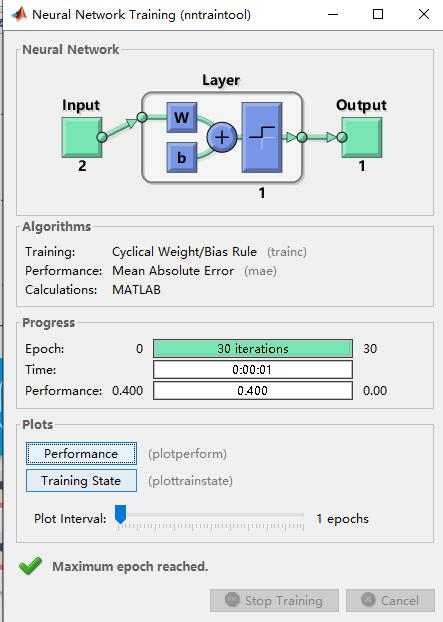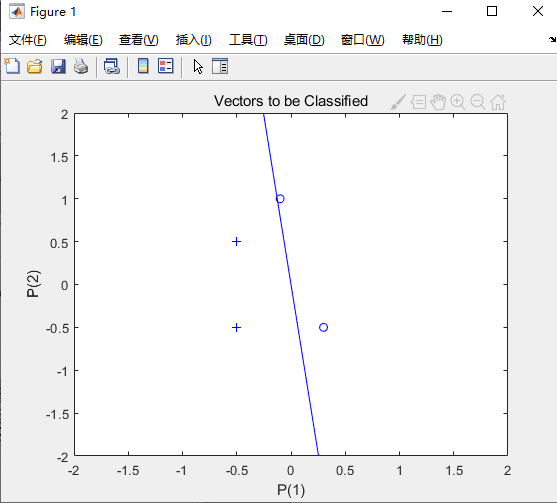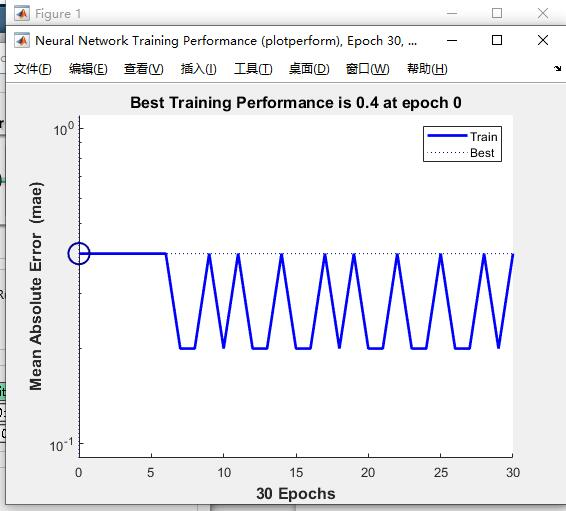BP神经网络代码实现
% BP网络
% BP神经网络的构建
net=newff([-1 2;0 5],[3,1],{'tansig','purelin'},'traingd')
net.IW{1}
net.b{1}

p=[1;2];
a=sim(net,p)
net=init(net);
net.IW{1}
net.b{1}
a=sim(net,p)
%net.IW{1}*p+net.b{1}
p2=net.IW{1}*p+net.b{1}
a2=sign(p2)
a3 = tansig(a2)
a4=purelin(a3)
net.b{2}
net.b{1}

net.IW{1}
net.IW{2}
0.7616+net.b{2}
a-net.b{2}
(a-net.b{2})/ 0.7616
help purelin

p1=[0;0];
a5=sim(net,p1)
net.b{2}
% BP网络
% BP神经网络的构建
net=newff([-1 2;0 5],[3,1],{'tansig','purelin'},'traingd')
net.IW{1}
net.b{1}
%p=[1;];
p=[1;2];
a=sim(net,p)
net=init(net);
net.IW{1}
net.b{1}
a=sim(net,p)
net.IW{1}*p+net.b{1}
p2=net.IW{1}*p+net.b{1}
a2=sign(p2)
a3=tansig(a2)
a4=purelin(a3)
net.b{2}
net.b{1}


P=[1.2;3;0.5;1.6]
W=[0.3 0.6 0.1 0.8]
net1=newp([0 2;0 2;0 2;0 2],1,'purelin');
net2=newp([0 2;0 2;0 2;0 2],1,'logsig');
net3=newp([0 2;0 2;0 2;0 2],1,'tansig');
net4=newp([0 2;0 2;0 2;0 2],1,'hardlim');

net1.IW{1}
net2.IW{1}
net3.IW{1}
net4.IW{1}
net1.b{1}
net2.b{1}
net3.b{1}
net4.b{1}

net1.IW{1}=W;
net2.IW{1}=W;
net3.IW{1}=W;
net4.IW{1}=W;

a1=sim(net1,P)
a2=sim(net2,P)
a3=sim(net3,P)
a4=sim(net4,P)

init(net1);
net1.b{1}

help tansig

训练
% 训练
p=[-0.1 0.5]
t=[-0.3 0.4]
w_range=-2:0.4:2;
b_range=-2:0.4:2;

ES=errsurf(p,t,w_range,b_range,'logsig');%单输入神经元的误差曲面
plotes(w_range,b_range,ES)%绘制单输入神经元的误差曲面
pause(0.5);
hold off;
net=newp([-2,2],1,'logsig');
net.trainparam.epochs=100;
net.trainparam.goal=0.001;
figure(2);
[net,tr]=train(net,p,t);
title('动态逼近')
wight=net.iw{1}
bias=net.b
pause;
close;
% 练
p=[-0.2 0.2 0.3 0.4]
t=[-0.9 -0.2 1.2 2.0]
h1=figure(1);
net=newff([-2,2],[5,1],{'tansig','purelin'},'trainlm');
net.trainparam.epochs=100;
net.trainparam.goal=0.0001;
net=train(net,p,t);
a1=sim(net,p)
pause;
h2=figure(2);
plot(p,t,'*');
title('样本')
title('样本');
xlabel('Input');
ylabel('Output');
pause;
hold on;
ptest1=[0.2 0.1]
ptest2=[0.2 0.1 0.9]
a1=sim(net,ptest1);
a2=sim(net,ptest2);

net.iw{1}
net.iw{2}
net.b{1}
net.b{2}


MATLAB运行结果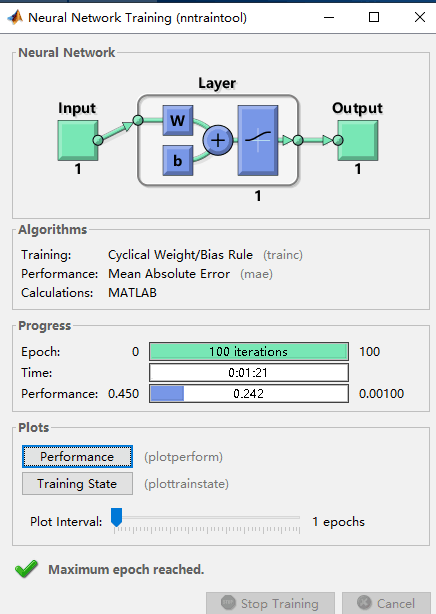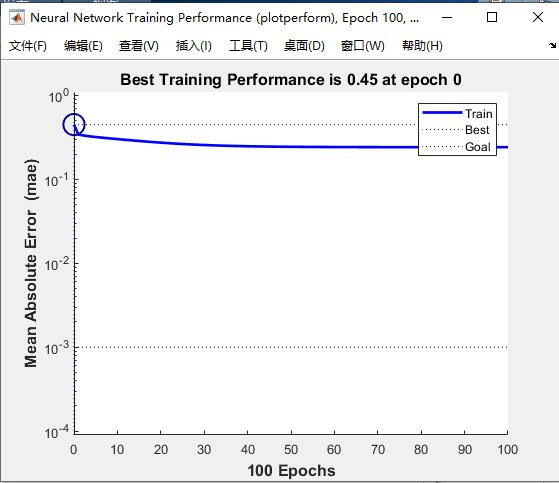单输入神经元的误差曲面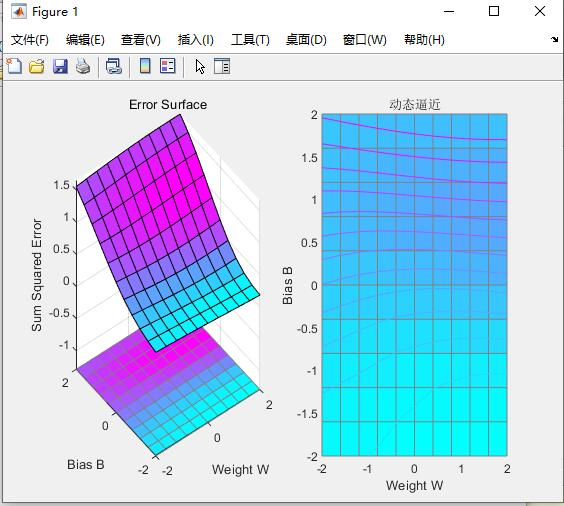展开全文• ## 感知机-BP神经网络

千次阅读 2017-01-04 00:41:06
感知机（perceptron）数据集的线性可分： 对数据集 若存在某个超平面 w·x+b=0，能够将数据集的正实例点负实例点完全正确地划分到超平面的两侧，即对所有y=+1的实例，w·x+b>0，对所有y=-1的实例，w·x+b，则认为...机器学习
• 文章目录神经元常见的激活函数输出层激活函数感知机感知机学习损失函数感知机学习算法感知机拓扑结构多层前馈神经网络神经网络的学习：误差逆传播算法（BP算法）标准BP算法累积BP算法BP网络的过拟合BP网络的全局极小...
• 感知机是用于二分类的线性分类模型，其输入是实例的特征向量，输出是实例的类别，类别取+1-1二个值，+1代表正类，-1代表负类。 如上图的圆圈就代表一个感知器。它接受多个输入（x1，x2，x3…），产生一个输出...
• 导读：这是《神经网络和深度学习简史》第一部分。这一部分，我们会介绍1958年感知机神经网络的诞生，70年代人工智能寒冬以及1986年BP算法让神经网络再度流行起来。 序言：深度学习掀起海啸机器学习 人工智能 深度学习
• 在前一章节（https://blog.csdn.net/u012132349/article/details/86166324），我们介绍了感知机可以实现与门... 今天我们将介绍感知机的局限性(严格说是单层感知机的局限性)。这里我们想用感知机实现异或门，所谓异...机器学习 深度学习 Python 人工智能
• 反之，如果不是线性的，可达到的效果远远不及线性数据的效果，曾经感知机因为不能处理异或问题，而被人批判，导致神经网络的研究停滞了几十年。 2.1、形式 感知机就是为了确定一条直线WX+b，我们这里引入了sign...
• @(人工智能)BP神经网络推导–通过误差传递学习内部表达这是研一学习一门课程的课程实践，当时另一位实验室小伙伴合作完成BP神经网络的推导以及部分应用，参考的是一本外文书籍（影印版）的部分章节，有我自己翻译...人工智能
• 在这些地方，所为的“BP神经网络”应该是多层感知机“MLP”。 他们不好奇吗？ 或许是习惯了吧。习惯创造语言。 在google搜索“Back-Propagation Neural Networks”，似乎这个不像是一个有比较广泛应用的短语。...
• 3.3 ReLU函数 4、小结 三、误差反向传播算法及BP神经网络 1、误差反向传播算法 2、梯度下降法 2.1 梯度下降法的数学推导 2.2 多层感知机的数学推导 3、BP神经网络具体实现 3.1 BP神经网络的实现步骤如下： 3.2 BP...算法 python 机器学习 人工智能
• 最近实验室师弟上这门课，老师布置了一个手写神经网络的作业，想想自己之前的实验都用的人家的框架，干脆这次自己也花点时间拿着个作业练练手。 我实现的就是一个非常简单的网络结构，网络层数可以随意加。 网络层...
• 感知机与感知器神经网络 感知器是人工神经网络中的一种典型结构。 Frank Rosenblatt在1957年就职于Cornell航空实验室(Cornell Aeronautical Laboratory)时所发明的一种人工神经网络。它可以被视为一种最简单形式的...
• （2）多层神经网络（解决线性不可分问题——在感知机神经网络上多加一层，并利用“后向传播”（Back-propagation）学习方法，可以解决XOR问题）（1974年，哈佛博士论文） （3）BP算法训练的神经网络：信号正向...
• 前馈神经网络由三部分组成：输入层（第0层），输出层（最后一层），中间部分称为隐藏层，隐藏层可以是一层，也可以是多层 。FNN如下图所示，其中圆圈是神经元。 学习神经网络前向传播与反向传播推导过程必须了解，...
• 1）前馈神经网络 (feedforward neural network)，又称作深度前馈网络(deep feedforward network)、多层感知机(multilayer perceptron，MLP) 顾名思义，该网络中没有反向传播反馈，因此无法自动修改网络参数。 2）全...深度学习
• 全连接网络（FC）、前馈神经网络BP）、多层感知机（MLP）三种称呼实质是一样的。以全连接网络为例讲解训练过程。 全连接网络实际上可以看作由多个隐藏层(dense)组成的网络模型，隐藏层的每个节点都与上一层所有...
• @(Aaron)[机器学习 | 多层感知机] 主要内容包括： 多层感知机的基本知识 使用多层感知机图像分类的从零开始...在这里，我们将以多层感知机（multilayer perceptron，MLP）为例，介绍多层神经网络的概念。   多层感知机器学习 深度学习 python 算法
• 这篇博客主要总结多层感知机（MLP）的一些知识要点，MLP也就是DNN（深度神经网络），是深度学习的基础。（原创 https://blog.csdn.net/baidu_33718858/article/details/84972537） 主要的参考文献来自于： ...MLP
• 通过合适的例子讨论多层感知机的运作 讨论利用反向传播bp的方法推导权值更新公式 课堂笔记 神经网络概念 神经网络（artificial neural network）是与人类大脑思考相似的算法，可用来解决监督式的分类...机器学习 分类 聚类
• 概念理解 感知机 感知机（perceptron）是二分类的线性分类模型，输入为实例的特征向量，输出为实例的类别（取+1-1）。感知机对应于输入空间中将实例划分为两类的分离超...多层感知机 多层感知器（Multilayer P...pytorch
• 多层感知机监督训练在线学习的流行正是由于反向传播（BP算法）算法的提出而得到了加强，BP算法可以说是神经网络的核心算法。  如下图所示，神经元j被它左边的一层神经元产生的一组函数信号所馈给。因此神经元j的...机器学习 MLP
• 多层感知机(MultiLayer Perceptron)以及反向传播算法(Backpropagation) 机器学习——神经网络（四）：BP神经网络 如果还不清楚这里有视频：PyTorch深度学习实践（强烈推荐！！讲的太清了，还讲了pytorch的代码。） ......